# H.1 Recursive Algorithm to Calculate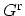The DYSON equation for the retarded GREEN's function and the left-connected GREEN's function  are employed to calculate the diagonal blocks of the full GREEN's function recursively. The solution to the matrix equation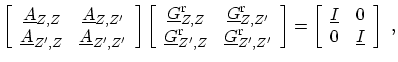(H.4)

is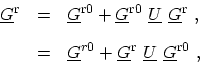(H.5)

where,(H.6)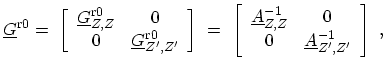(H.7)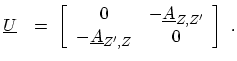(H.8)

The left-connected retarded GREEN's function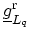is defined by the first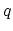blocks of (H.1) by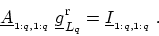(H.9)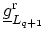is defined in a manner identical toexcept that the left-connected system is comprised of the first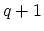blocks of (H.1). In terms of (H.4), the equation governingfollows by setting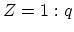and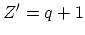. Using the DYSON equation [(H.5)], one obtains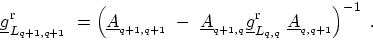(H.10)

It should be noted that the last block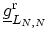is equal to the fully connected GREEN's function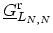, which is the solution to (H.1). The full GREEN's function can be expressed in terms of the left-connected GREEN's function by considering (H.4) such that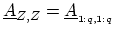,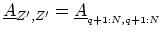and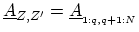. By noting that the only non-zero block of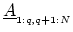isand using (H.5), one obtains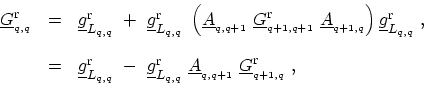(H.11)

Both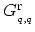and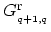are used for the calculation of the electron density, and so storing both sets of matrices will be useful. In view of the above equations, the algorithm to compute the diagonal blocksis given by the following steps
•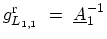,
• For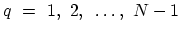, (H.10) is computed,
• For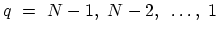, (H.11) is computed.
M. Pourfath: Numerical Study of Quantum Transport in Carbon Nanotube-Based Transistors